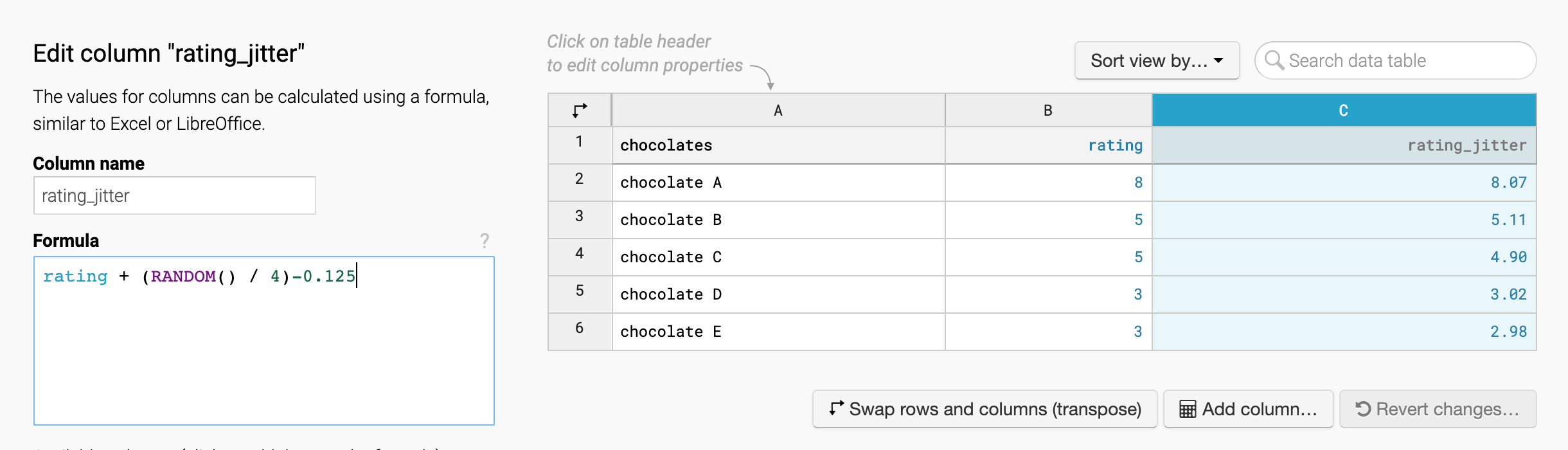# How to calculate in added columns

When creating a chart, you have the option to add a column in step 2: Check & Describe: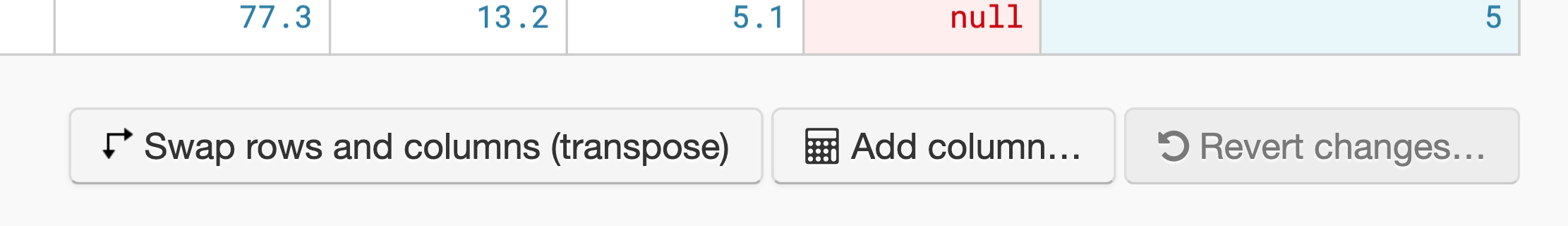Added columns are perfect to perform calculations on your uploaded columns. For example, you can sum up numbers, create conditions, or trim text. In this example, we multiply the data from two columns with each other and name this new column "GDP":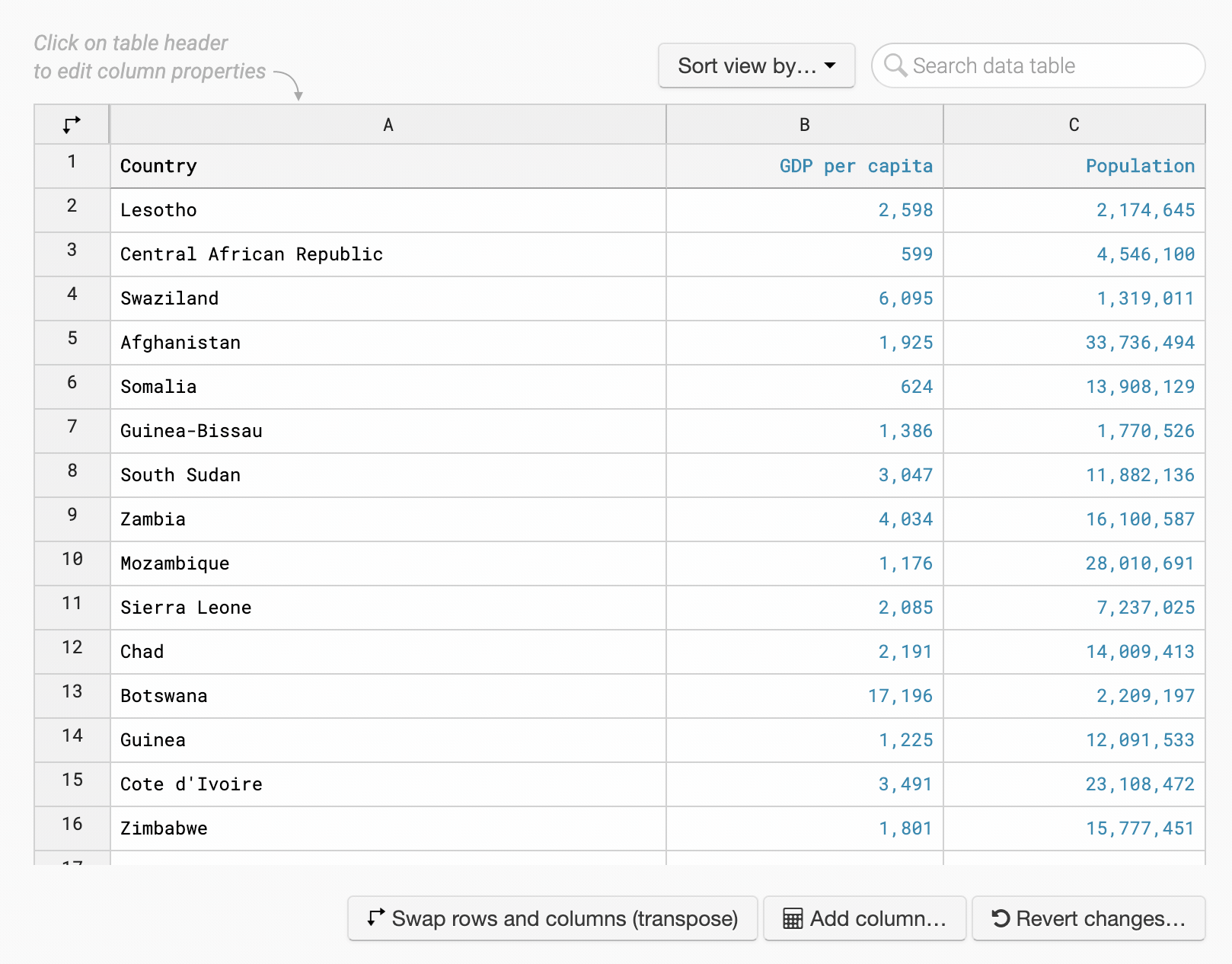This article explains which expressions are allowed. They will remind you of Excel, LibreOffice or Google Sheets formulae – and you're right, we took some inspiration. You can also always prepare the final data before uploading it to Datawrapper.

At the end of the article, you find a few more examples to give you a better understanding of what's possible.

## Values

You can use the following values in your formulae:

• numbers, e.g. `3` or `3.14`
• text, e.g. `"quick brown fox"`
• column names, e.g. `gpd_per_capita`
Then you can use various expressions to process these values.
👉 The following tables show just a selection of the possible expressions. You can find an always up-to-date, complete list in this Github README.

## Expressions for numbers

Expression Explanation Example
+ - * / % ^ simple math operators (use parentheses for grouping) `value ^ 2` or `(value + 3) * 5`
ABS absolute number `ABS(value)` or `ABS value`
SQRT square root `SQRT(value)` or `SQRT value`
SIN, COS, TAN, ASIN, ACOS, ATAN trigonometric functions `COS(value)`
LOG, LOG2, LN, LG, LOG10, LOG1P logarithmic functions `LOG10(value)`
MIN, MAX minimum / maximum `MAX(value, foo, 42)`
SUM sum `SUM(value, foo, 42)`
MEAN, MEDIAN returns the median or mean of the given numbers
`MEAN(y, x)`
ATAN2 calculate angle ( Wikipedia) `ATAN2(y, x)`
SIGN sign of number; returns 1 if number is positive, -1 if number is negative `SIGN(value)`
ROUND round number to decimal `ROUND(value)` or `ROUND(value, decimals)`
FLOOR, CEIL, TRUNC other ways of rounding `FLOOR(value)`
PI, E constants ` PI * value`
NUMBER convert text to number `NUMBER(text)` or `NUMBER text`
RANDOM generate a random real number between 0 and 1 when used without arguments, or between 0 and the passed number `RANDOM()` or `RANDOM(10)`

## Expressions for text

Expression Explanation Example
LOWER lowercase a string `LOWER("Hello World")` > hello world
UPPER uppercase a string `UPPER("Hello World")` > HELLO WORLD
TITLE convert a string to title-case. Like  `PROPER`, but better for headlines.
`TITLE("IDA lloyd-webber")` > Ida Lloyd-webber
PROPER convert a string to title-case. Like  `TITLE`, but better for names. `PROPER("IDA lloyd-webber")` > Ida Lloyd-Webber
LENGTH length of a text `LENGTH name` or `LENGTH(name)`
TRIM remove whitespace from the beginning and end of text `TRIM(name)` or `TRIM name`
SUBSTR extract substring from text `SUBSTR(name, start, length)`
CONCAT concatenate multiple texts `CONCAT(firstname, " ", lastname)`
SPLIT split text into parts by a substring
`SPLIT(name, " ")`
JOIN join parts back into text with a glue string (e.g. a comma). You can define a second glue for the last join (e.g. "and").
`JOIN(parts, ",")`
REPLACE replaces all occurrences of substring in text `REPLACE(country, "United States", "USA")`
REPLACE_REGEX like REPLACE, but interprets the search string as regular expression
`REPLACE_REGEX("hello 123 world", "[0-9]", '#')`
TEXT convert number to text `TEXT(value)` or `TEXT value`

## Expressions for dates

Expression Explanation Example
DATE get a current date; or create date from passed year, month and day values (the month parameter is interpreted as 1 = January, 2 = February...); or create date from date as text
`DATE()` or `DATE(2020, 5, 1)` or `DATE(text)`
YEAR extract year from date `YEAR(date)`
MONTH extract month from date (1 = January, 2 = February...) `MONTH(date)`
DAY extract day of the month from date `DAY(date)`
HOURS extract hours from datetime `HOURS(date)`
MINUTES extract minutes from datetime `MINUTES(date)`
SECONDS extract seconds from datetime `SECONDS(date)`
WEEKDAY day of the week (0 = Sunday, 1 = Monday...) `WEEKDAY(date)`
DATEDIFF calculate number of days between two dates `DATEDIFF(first_date, second_date)`
TIMEDIFF calculate number of seconds between two dates `TIMEDIFF(first_date, second_date)`

## Logical expressions

Expression Explanation Example
IF if-else statement `IF(condition, yay, nay)` or `condition ? yay : nay`
NOT, ! invert expression `NOT(condition)` or `NOT condition` or `!condition`
and logical and `condition1 and condition2`
or logical or `condition1 or condition2`
>, <, ==, <=, >=, != comparisons
in check if a substring is included in text; or check if a value is included in parts created by `SPLIT` `"John" in name` or `"John" in parts`
ROWNUMBER current rownumber, starting at 0 `ROWNUMBER` → 0, 1, 2, ...

## Aggregations

Each number column comes with aggregation values written like this: columnname_aggregation. For example, to get a column with the minimum value of your column "percent_change", insert "percent_change__min" into the formula field: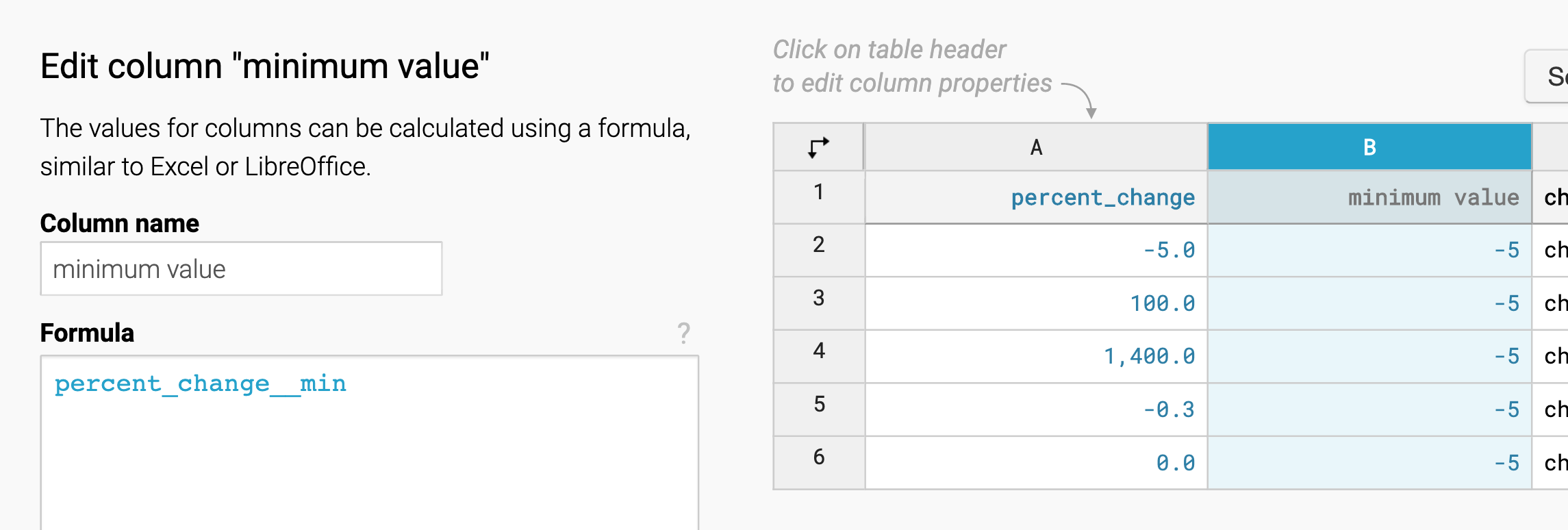You can also calculate with these values. Here's an overview of all available aggregations:

 Aggregation Explanation Example __min returns the minimum value in the column `gdp__min` __max returns the maximum value in the column `gdp__max` __sum returns the sum of all values in the column `gdp__sum` __mean returns the mean (= average) of all values in the column `gdp__mean` __median returns the median of all values in the column `gdp__median`

This can be helpful for conditions:
```IF(salary > salary__mean, "earns more than the average", "earns less than the average")
```

This gives you a column that shows whose salaries are greater or smaller than the average one.
__min and __max also work for dates. This is helpful, e.g., to find out how many days have passed since the date in your data that's furthest in the past:
```DATEDIFF(date__min, date)<br>
```

Let's look at some more examples of how to use these expressions:

## Example 1: "pos./neg." with IF

Let's say you have a table with percentage changes, like -5% or +100%. It's sufficient for your reader to know if the change is positive or negative, so you want to print a column that says "pos." or "neg." depending on the change. To do so, add a new column and use the following formula:

```IF(percent_change > 0, "pos.", "neg.")
```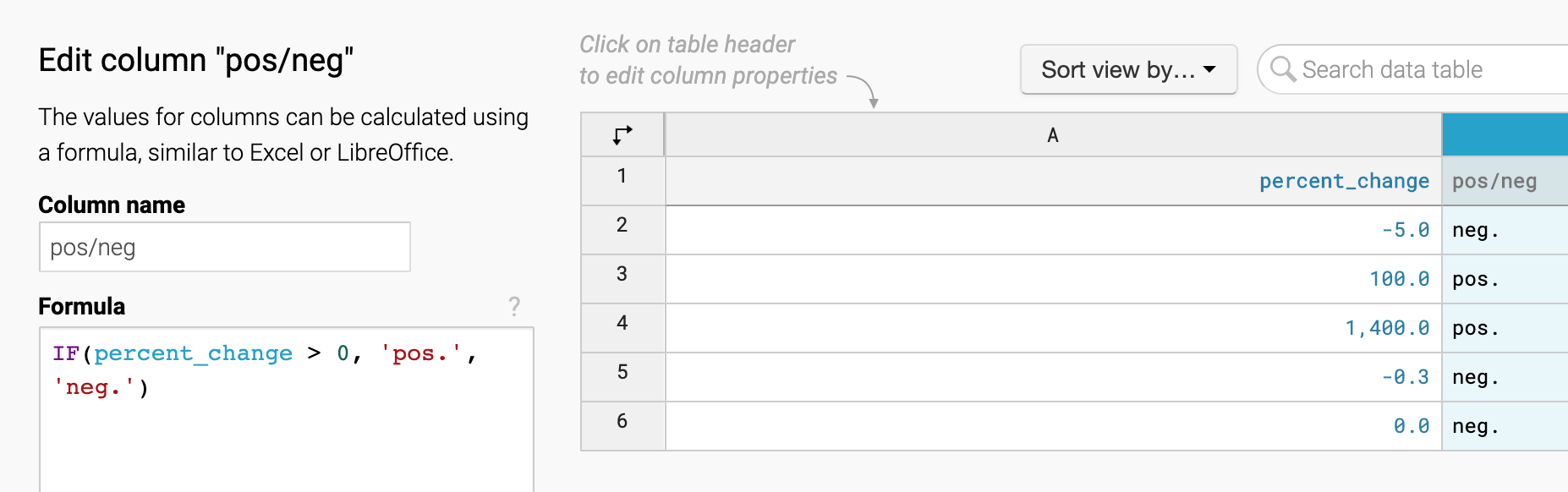## Example 2: Line breaks with JOIN/SPLIT

Let's say you uploaded a column with country names. For your tooltips, you want more line breaks in your long country names. Add a new column and insert the following formula:

```JOIN(SPLIT(country, " "), "<br/>")
```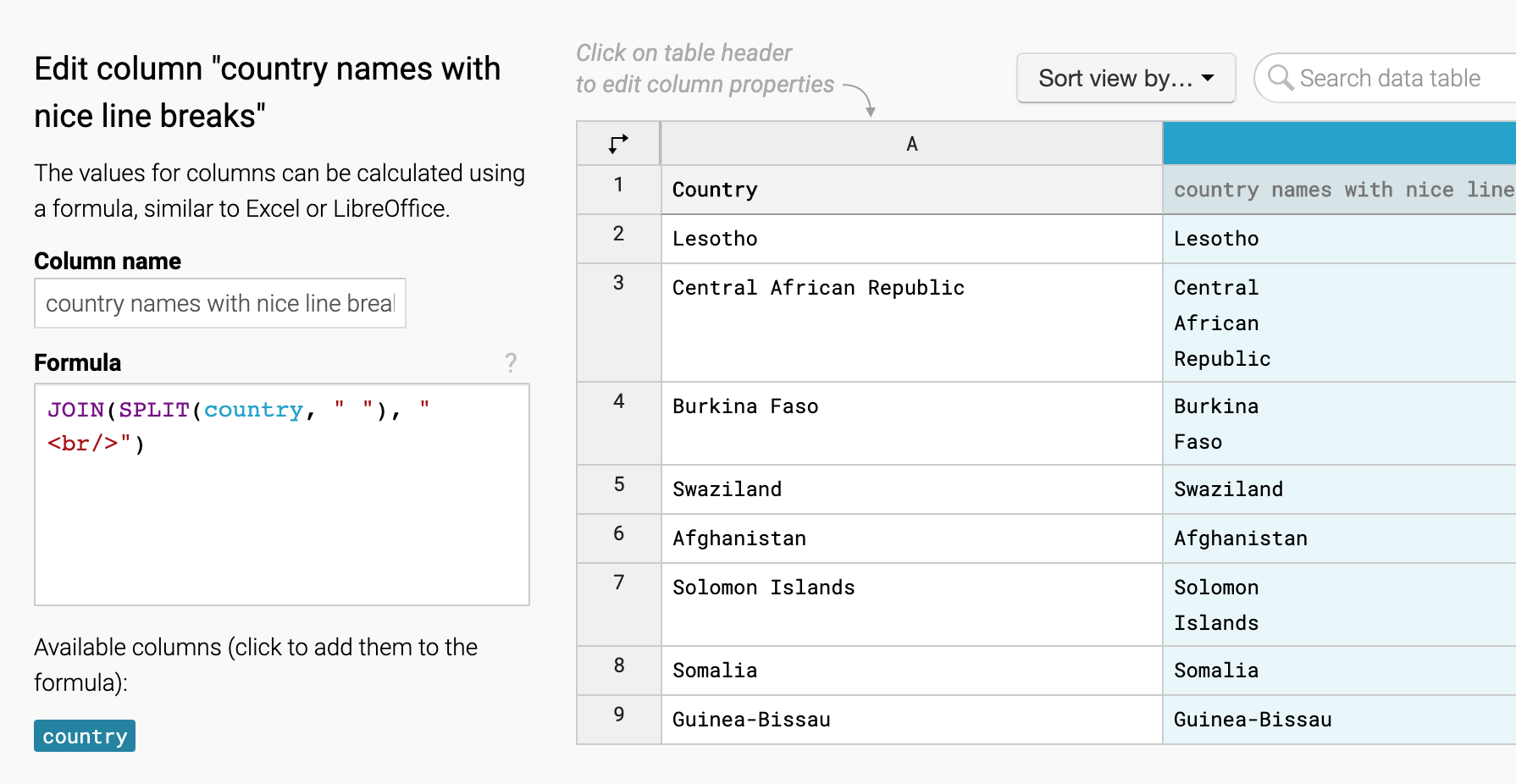You could also use REPLACE() – sometimes there are multiple ways to achieve something.

## Example 3: First names with SPLIT

SPLIT is also a good way to just get the first (second, third, etc.) words when you have multiple ones. Let's say you upload a list of the first and last names of The Beatles, but you just want their first names. Try the following formula:

```(SPLIT(the_beatles, " "))
```

This will take the first word (that's a position zero) of your split words: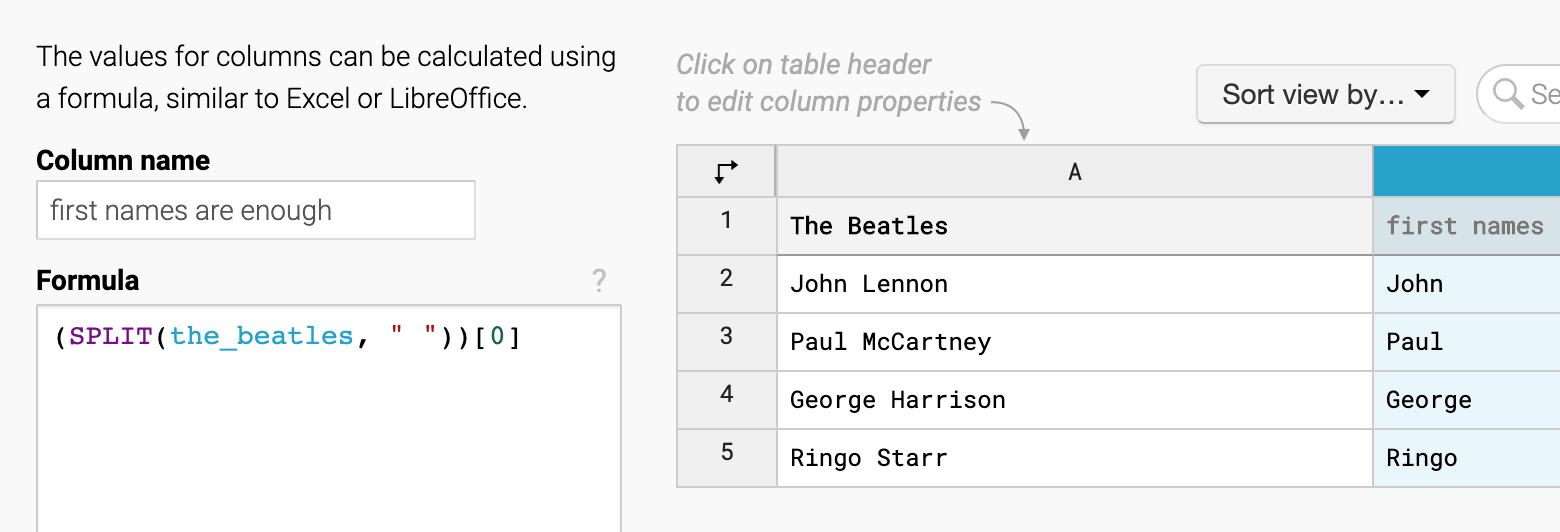## Example 4: Jitter with RANDOM()

Let's say you upload a dataset with chocolate rated with grades from 1-10. You want to display it on a scatterplot, but all the chocolates would then lay exactly on top of each other. (We wrote about this problem in this blog post.) It's time to introduce some jitter. To do so, use the following formula:

```rating + (RANDOM() / 4) - 0.125
```

This divides a random number between 0 and 1 (e.g. 0.4) by 4 (→ 0.1) and then subtracts 0.125 (→ -0.025):